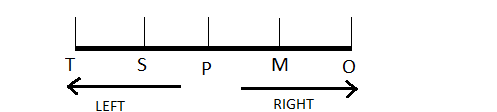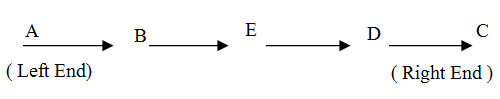## Reasoning :: Sitting ArrangementPage 1

###### Section-1

1.

 There are five different houses A to E, in a row. A is to the right of B and E is to the left of C and right of A, B is to the right of D. Which of the houses is in the middle? (a)A (b)B (c)C (d)D
D B A E C
So, the house in the middle is A.

2.

 A, B, C, D, E, and F are sitting in a row. E and F are in the centre and A and B are at the ends. C is sitting on the left of A. Then who is sitting on the right of B? (a)A (b)D (c)E (d)F
Answer is: BAccording to the question, sitting arrangement is at follow.

B D E/F F/E C A

Clearly, D it sitting on the right of B.

3.

 At a Birthday Party, 5 friends are sitting in a row. M is to the left of O and the right of P. S is sitting to the right of T but to the left of P. Who is sitting in the middle? (a)M (b)O (c)P (d)S
Answer is: CSo, P is sitting in the middle.

4.

 In a gathering seven members are sitting in a row. C is sitting left to B but on the right to D. A is sitting right to B. F is sitting right to E but left to D. H is sitting left to E. Find the person sitting in the middle? (a)C (b)D (c)E (d)F
Answer is: BAccording to the question,

arranging the seven members in a row.

H E F D C B A

Hence, D is sitting in the middle.

5.

 Five friends P, Q, R, S and T are sitting in a row facing north. Here S is between T and Q and Q is to the immediate left of R. P is to the immediate left of T. Who is in the middle? (a)S (b)T (c)Q (d)R
Answer is: AAccording to the questions, sitting arrangement of all the five friends is as follow, P T S Q R Hence, S is sitting in the middle.

6.

 Five boys A, B, C, D and E are standing in a row. D is on the right of E. B is on the left of E but on the right of A, B is on the left of C, who is standing on the extreme right. Who is standing in the middle? (a)B (b)C (c)D (d)E
Answer is: DThe sequence of boys in a queue isSo, E is sitting in the middle.

7.

 Six friends A, B, C, D, E and F are sitting in a row facing towards North. C is sitting between A and E, D is not at the end, B is sitting at immediate right of E, F is not at the right end, but D is sitting at 3rd left of E. Which of the following is sitting to the left of D? (a)A (b)F (c)E (d)C
Answer is: BAccording to the question, arrangement of A, B, C, D, E and F is as follows
F D A C E B
Thus, F is sitting to the left of D.

8.

 A, P, R, X, S and Z six persons sitting in a row. S and Z are at the centre. A and P are at the ends. R is sitting on the left of A. Then, who is sitting on the right of P? (a)A (b)S (c)X (d)Z
Answer is: CGiven figure shows the correct positions of six persons.Hence, it is clear that X is on the right of P.